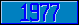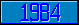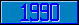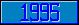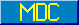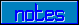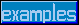## \$%CSCH

### M[UMPS] by Example

```
2-Jul–96, 6:54:35

Routine Save for Hyperbolic Trigonometry Library function \$%CSCH^MATH

;

; Unless otherwise noted, the code below

; was approved in document X11/95–13

;

; If corrections have been applied,

; first the original line appears,

; with three semicolons at the beginning of the line.

;

; Then the source of the correction is acknowledged,

; then the corrected line appears, followed by a

; line containing three semicolons.

;

CSCH(X,PREC) ;;;Quit 1/\$%SINH^MATH(X,PREC)

; Winfried Gerum (8 June 1995)

; Alan Frank (October 1995)

Quit 1/\$%SINH^MATH(X,\$Get(PREC,11))

;;;

;

;===

;

;

;

```